3D rasters

Usage: soil properties, concentrations in water bodies, properties of air mass, hyperspectral data, analysis of spatio-temporal data, forest and habitat properties, exploration of simulation scenario space, etc.

• 3D raster - Voxel space - 3D voxel grid - Voxel model
• [3D] cell - Voxel - 3D voxel unit - Volumetric pixel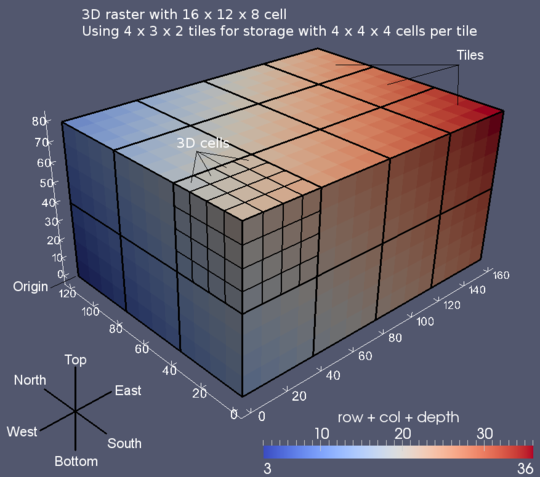Image credit: GRASS Development Team

Series of 2D rasters

• 2D does not explicitly represent and model 3D relationships
• 2D rasters accessible individually3D raster

• explicit 3D relationships
• needs to be sliced to get 2D rasters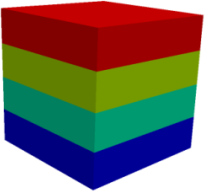Third dimension

• The first two dimensions are the same as in 2D.
• Third dimension can be in space, time, or scenario space.
• Mind the possible anisotropy in the Z direction.
(different from 2D rasters where all directions are isotropic most of the time)Working with 3D rasters

Not that different from 2D rasters, images, and matrices

Python

• using NumPy array
x = array([[[ 0,  1,  2],
[ 3,  4,  5],
[ 6,  7,  8]],
[[ 9, 10, 11],
[12, 13, 14],
[15, 16, 17]],
[[18, 19, 20],
[21, 22, 23],
[24, 25, 26]]])
x.sum(axis=0)
# array([[27, 30, 33],
#        [36, 39, 42],
#        [45, 48, 51]])R

• using array
x = array(1:40, dim=c(2,4,5))
# , , 1
#
#      [,1] [,2] [,3] [,4]
# [1,]    1    3    5    7
# [2,]    2    4    6    8
#
# , , 2
# ...
x[2,3,3]
# 22
x[2,3,3] = 0Octave

• using 3D matrix/array
x = reshape(1:8, 2, 2, 2)
a(2,1,1) = 5
b = 3 * a
# b =
#
# ans(:,:,1) =
#
#     3    9
#    15   12GRASS GIS

general-purpose geospatial analysis and remote sensing tool

• data types:
• vectors
• 2D rasters
• 3D rasters
• container types:
• imagery groups
• series of 2D rasters
• spatio-temporal datasets
• series of 2D rasters (or vectors or 3D rasters)Moving window

2D

r.neighbors input=a output=b method=average size=5
r.colors map=b color=viridis

3D

r3.neighbors input=a output=b method=average window=5,5,5
r3.colors map=b color=viridis

3D raster algebra

b = a + 2
Conditional expression
b = if(a > 20, 1, null())
Accessing neighboring cells
b = (a[0,0,-1] + a[0,0,1]) / 2

Visual programming

• graphical modeler
• graphical raster calculator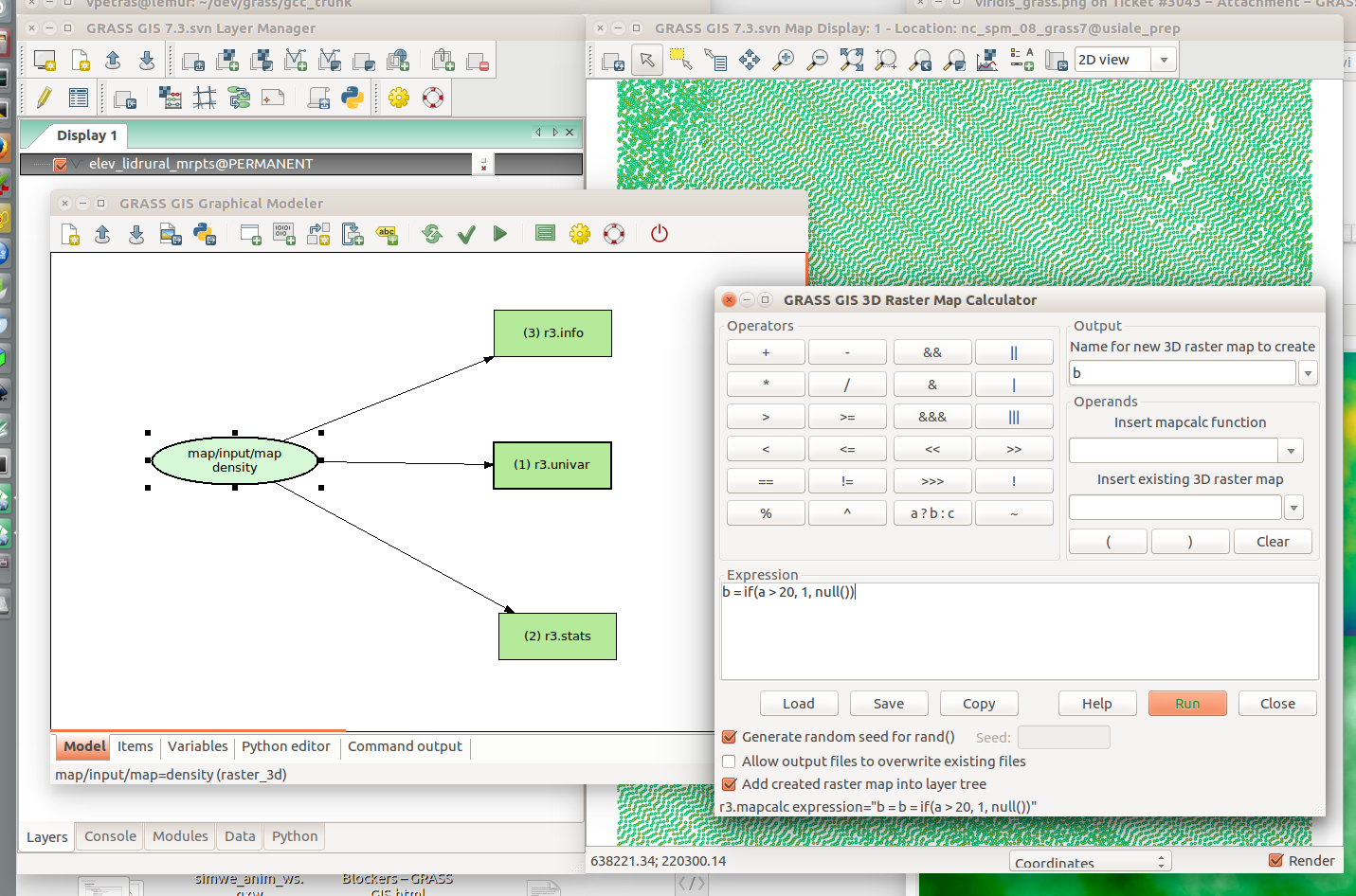Example: Terrain evolution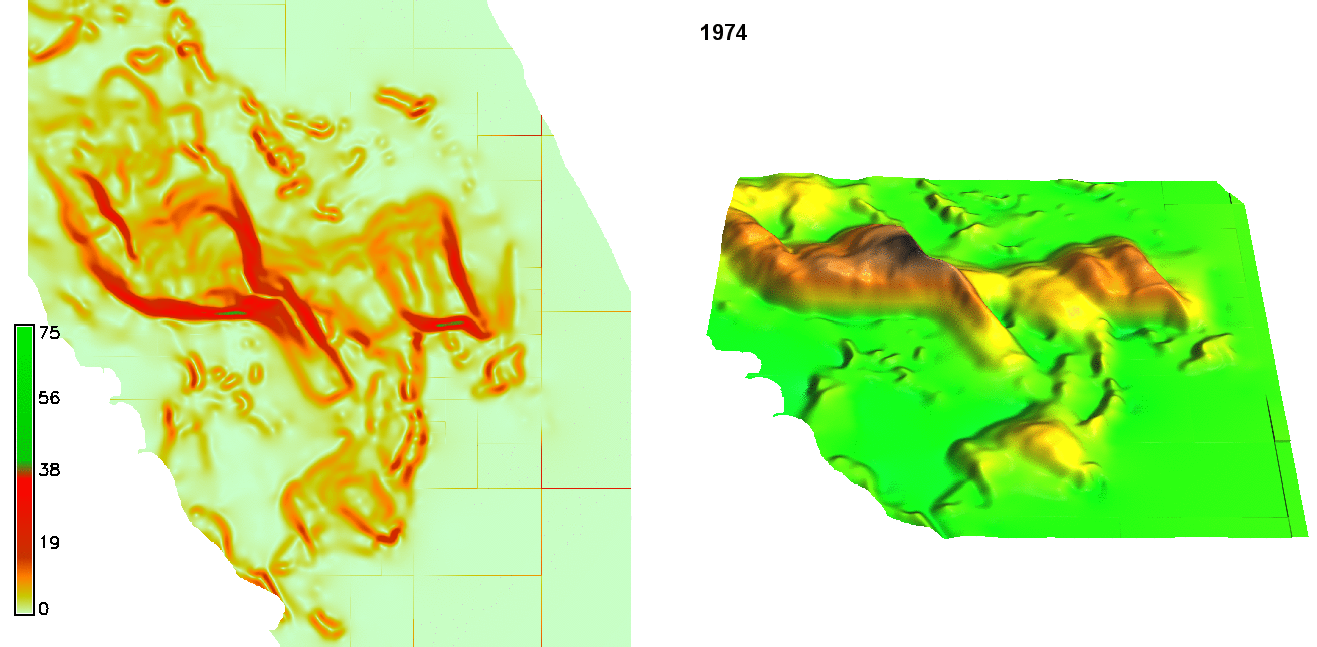Example: Terrain evolution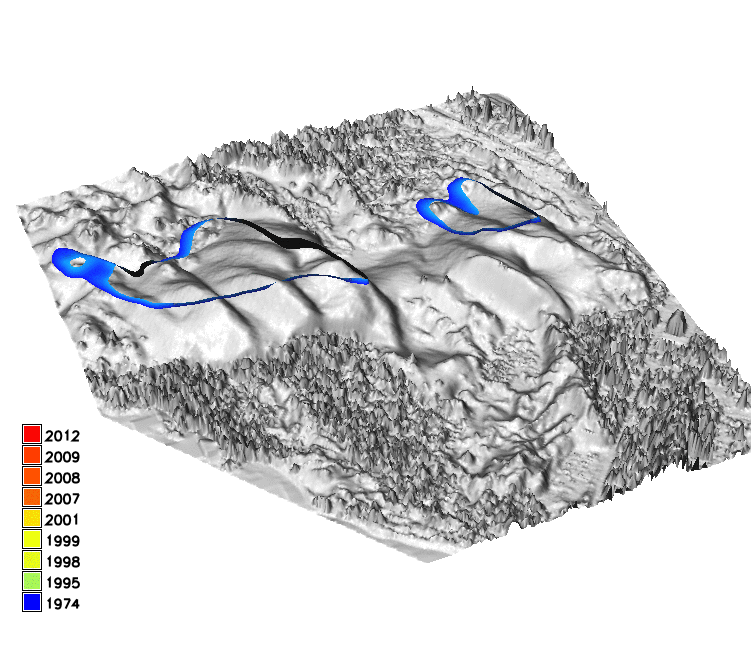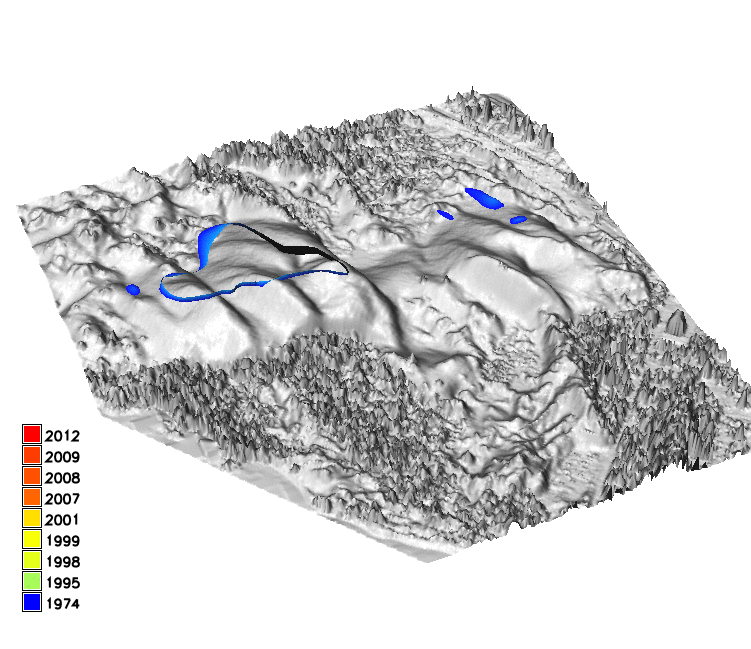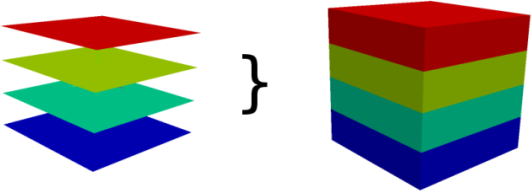Image credit: GRASS Development Team

Example: Behavioral patterns

presence of people over time in camera view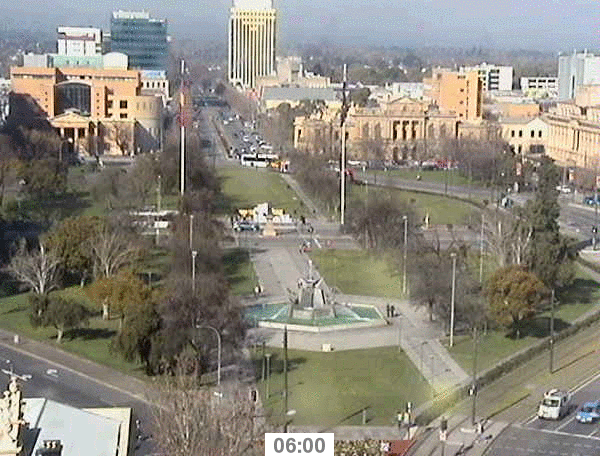Petrasova A., Hipp, A.J., Mitasova, H., Using space-time cube for visualization and analysis of active transportation patterns derived from public webcams. ICC 2017, Washington DC 2-7th July

Example: Behavioral patterns

spatio-temporal data representing movement of people in urban environment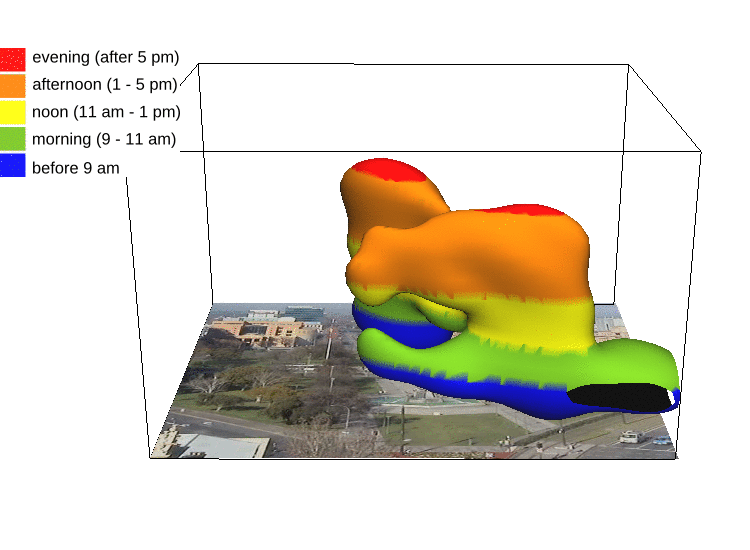Petrasova A., Hipp, A.J., Mitasova, H., Using space-time cube for visualization and analysis of active transportation patterns derived from public webcams. ICC 2017, Washington DC 2-7th July

Example: Vegetation structure

3D vegetation structure derived from lidar point clouds Petras, V., D. J. Newcomb, and H. Mitasova. Generalized 3D fragmentation index derived from lidar point clouds. In: Open Geospatial Data, Software and Standards [in print]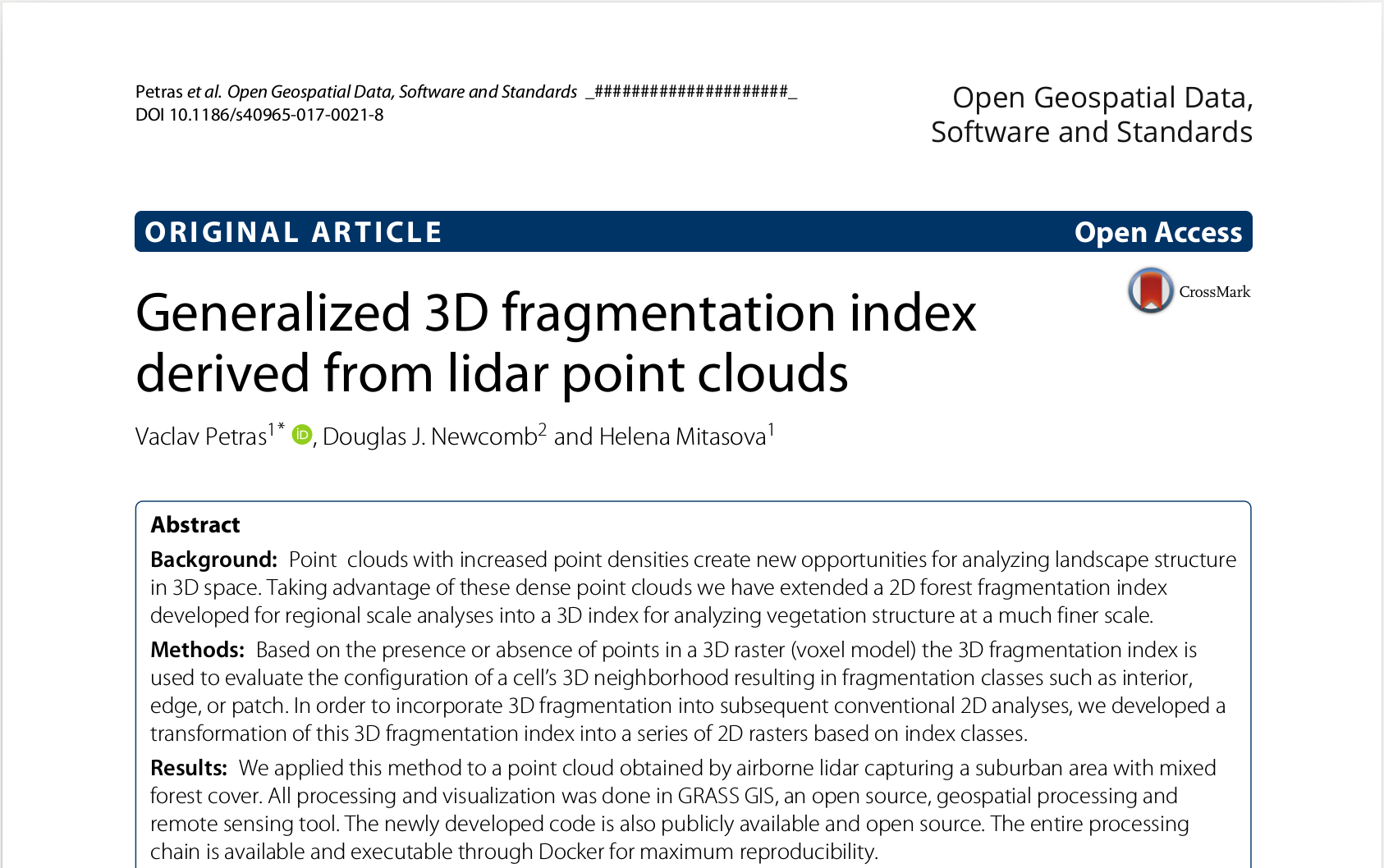3D version of forest fragmentation index by Riitters et al.

3D index of 3D raster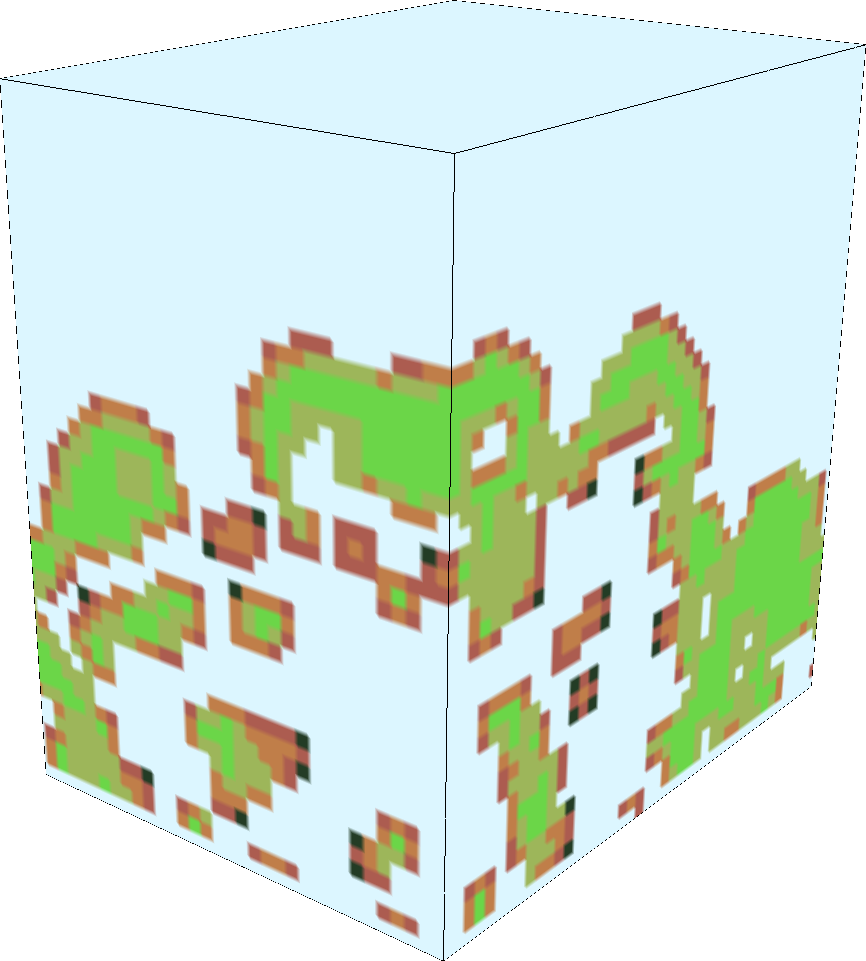Petras et al. 2017 [in print]

Point cloud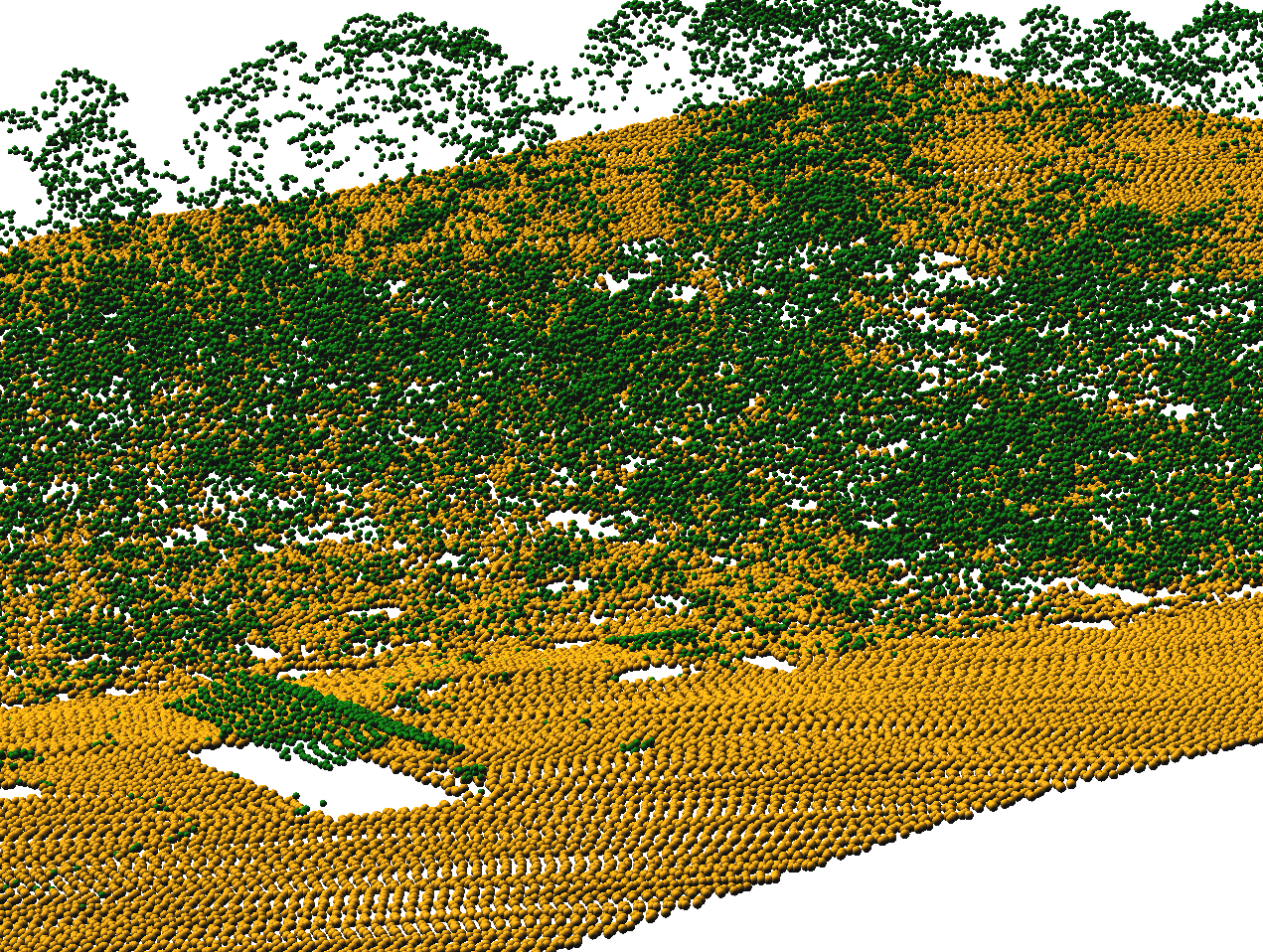Petras et al. 2017 [in print]

Binning, moving window & raster algebra

# Bash
r3.in.lidar input=points.las n=count base_raster=ground -d class_filter=3,4,5
r3.neighbors input=count output=average method=average window=3,3,3
r3.mapcalc expression="buffer = if(average > 0, 1, 0)"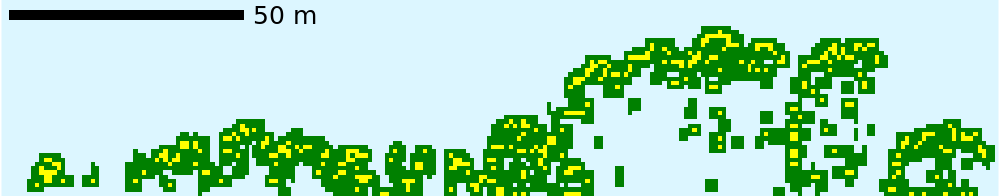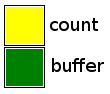Petras et al. 2017 [in print]

Fragmentation index

# Python
import grass.script as gs
gs.run_command("g.extension", extension="r3.forestfrag")
gs.run_command("r3.forestfrag", input="buffer", output="fragmentation")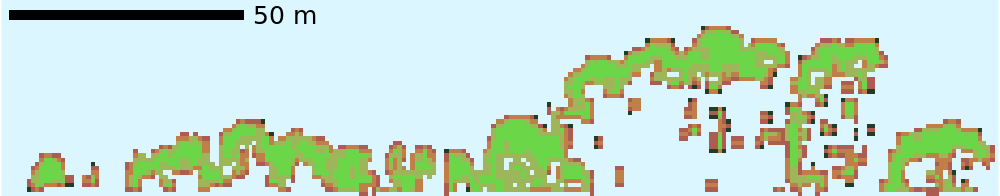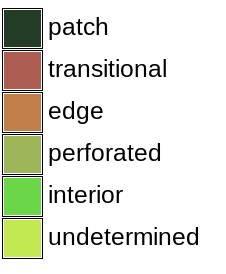Petras et al. 2017 [in print]

Profile of 3D raster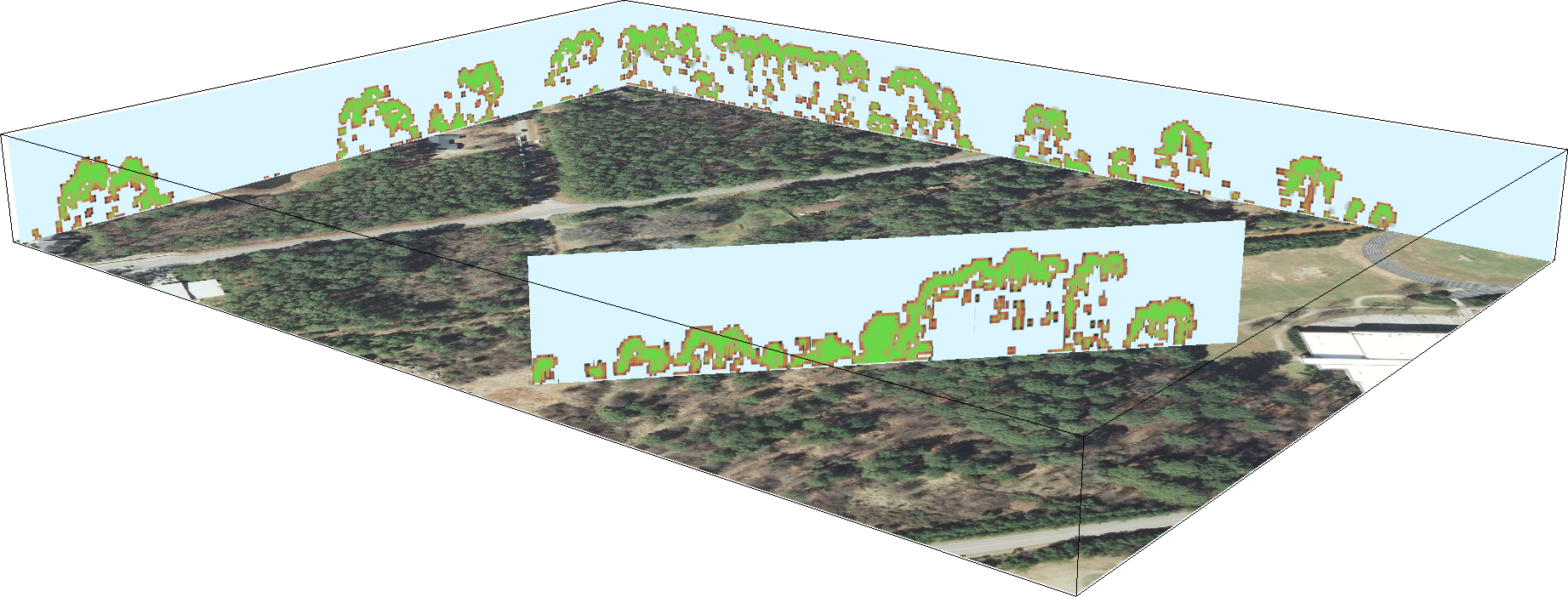Petras et al. 2017 [in print]

Back to 2D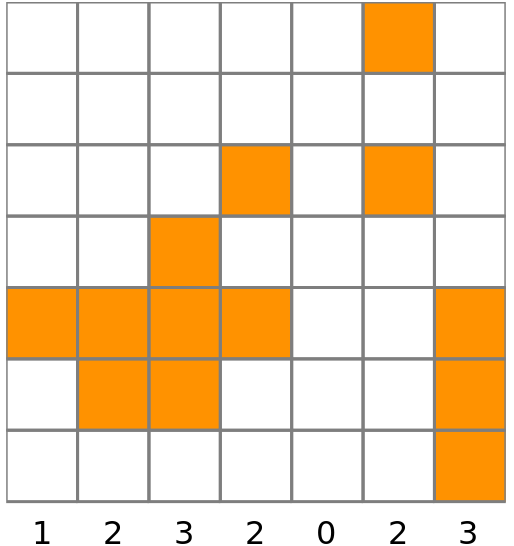# Bash
g.extension r3.count.categories
r3.count.categories input=fragmentation output=count
g.list type=raster pattern="count_*"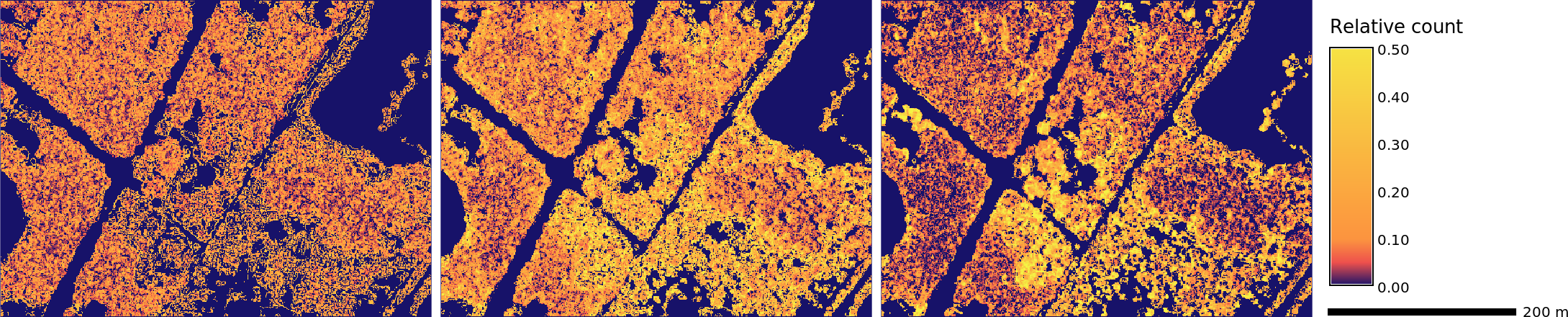Petras et al. 2017 [in print]

Conclusion

• there is a third dimension
• 3D rasters can handle it
• use the tool you know, or pick oneGRASS GIS grass.osgeo.orgPython python.orgR r-project.orgOctave octave.org